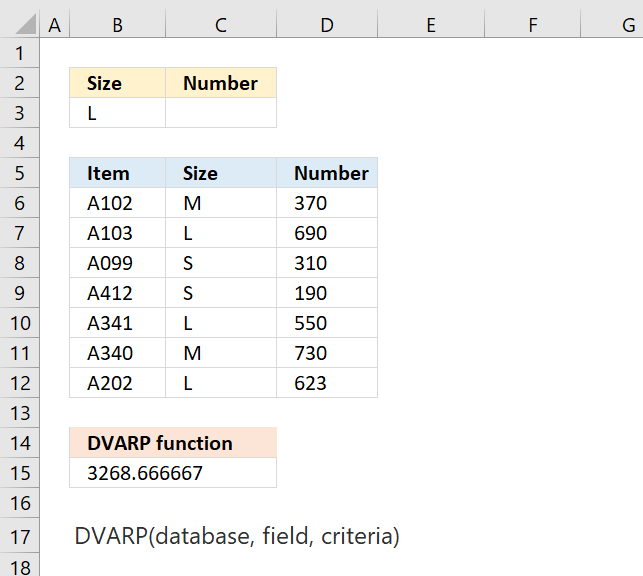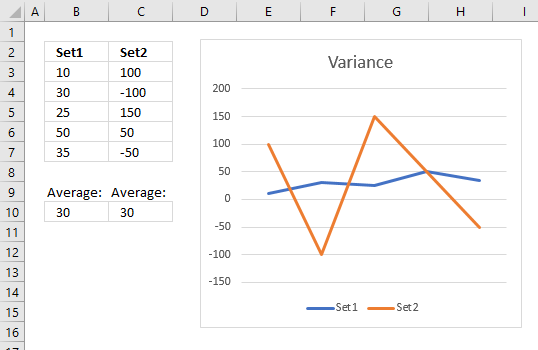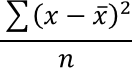Author: Oscar Cronquist Article last updated on May 05, 2022The DVARP function returns the variance of an entire population. The numbers are in a column of records in a dataset or database that meets a given condition or criteria.

Formula in cell B15:

=DVARP(B5:D12, 3, B2:D3)

The formula calculates the variance of the records that match condition Size "L". The corresponding numbers in column D are 690, 550 and 623.

Variance shows how much a set of numbers are spread out from their average value, see below example.Both Set1 and Set2 above have the same average 30, however, values in Set2 are much more spread out.

Set1 variance: 170 and Set2 variance: 8600.

### Excel Function Syntax

DVARP(database, field, criteria)

### Arguments

 database Required.  The cell reference to a list or database. field Required. The field argument lets you choose which column to use. You can use the column name enclosed with double quotation marks or the corresponding column number. criteria Required.  A cell reference to the criteria range. The criteria range needs to have column labels and at least one condition below the column label.

Excel defines a database as a list of related data in which rows of related information are records, and columns of data are fields. The first row of the list contains labels for each column.

Allowed criteria range characters are less than and greater than signs <>, use them to specify a criteria range. Also, asterisks * can be used to match partial strings.

You can place your criteria range wherever you want on your worksheet, however, it is not recommended below the list/database. The function needs a blank row below the list to work properly.

To include the entire list/database enter a blank line below the criteria range column labels.

The equation for DVARP is:x is the sample mean AVERAGE(number1,number2,…)

n is the sample size.